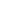# What Is A Loop?

Stripe Integration Freelance Gig🔗 See other jobs

## Definition

One of the beautiful things about a computer is its ability to follow a list of instructions from top to bottom, executing them at a fast pace.

But the most remarkable part is its ability to continue performing that same task over and over and only stops when it finds an answer for us based on a check we set.

## Use Cases and Examples

Now take the task of adding all natural numbers from `1` to `1000` thus `1,2,3,4,5,6...1000` this is something that will take us a while to do by hand, and will also take us some amount of time if we had to tell the computer "hey add 1 to 2 then to 3 all the way up to 1000".

Instead, we can tell the computer "look add numbers up and stop when you get to 1000". This is what makes loops a useful part of modern programming languages.

Here is an example in the Javascript programming language adding up numbers from 1 to 1000:

``````
02:     var sum = 0;
03:     for(var i = 1; i <= 1000; i++) { // here is the loop
04:       sum += i;
05:     }
06:     console.log("The sum is:", sum);
07: }
08:
09: adder(); //The sum is: 500500``````

In the example code above we have the line `for(var i = 1; i <= 1000; i++) {` which is the key part of our loop. It defines the start of the counting number as `1` using `var i = 1` and at the far end of it we have `i++`, which will keep increasing `i` by 1 and stop when `i` is greater than `1000` as represented by `i <= 1000`. The second important piece of all this is the line `sum += i`. `sum` is acting as a store or bucket of sorts (called a variable in programming) and its job is to add and store the numbers as the loop generates them.

Then in the end we print out the total number `sum` stored, this will be the total sum of `1` to `1000` thus `500500`.

## Summary

Loops are an integral part of software, it takes just a little bit more time for newer developers to learn and use them efficiently, this includes how to debug them when something goes wrong.

There are other ways to achieve the concept of looping without using the built-in syntax provided by the programming language, other programming languages also don't provide this syntax and require the developer to depend on concepts like recursion to achieve a looping effect.

Here is another article you might like 😊 "What Is A Macro?"